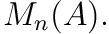### Basic facts about matrix algebras

Posted on May 25, 2019

Let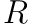be a ring, and let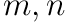be positive integers.

Proposition 1. The-algebras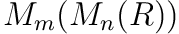and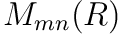are isomorphic.

The obvious map between is clearly a bijection and can be seen to be an-algebra homomorphism by observing that multiplication works as expected in each.

Proposition 2. If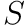is an-algebra, then there is a natural isomorphism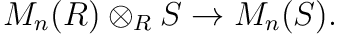Let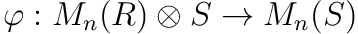be defined by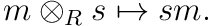This map is clearly an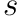-algebra homomorphism.

Let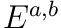be the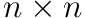matrix with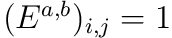if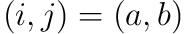and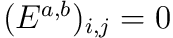otherwise. Observe that the map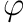is onto as thematrices generate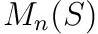as an-algebra.

Suppose that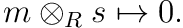Then either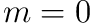,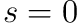, or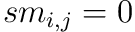for all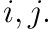Writing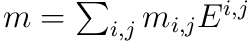we see thatThusis injective and hence an isomorphism.

Proposition 3. If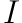is an ideal of, let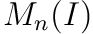denote the subset of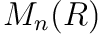consisting of matrices with entries in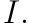The identification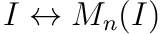is a bijection between the set of two-sided ideals of the ringand the set of two-sided ideals of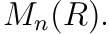First observe that ifis a two sided ideal ofthenas defined above is clearly a two-sided ideal ofNow suppose that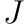is a two sided ideal ofand define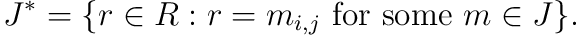It is easy to see that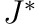is a two sided ideal ofsinceis a two sided ideal ofas follows. Suppose that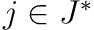and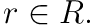By the definition ofthere exists a matrix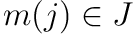with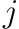as an entry. We then easily define a matrix with one nonzero entry equal to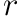such that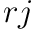is an entry of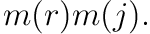Hence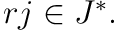A similar argument shows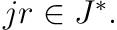It now suffices to show that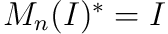and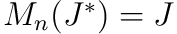for any two sided ideals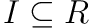and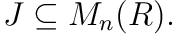It is immediate from definitions that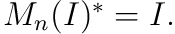To show the second equality, first observe that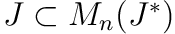by definition. For any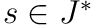, by matrix multiplication we can construct a matrix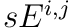(with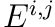defined as above). Hence we can generate all matrices in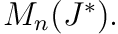we can construct a matrix

Proposition 4. Let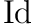denote the identity matrix inThe map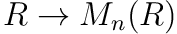defined by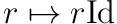identifieswith the centre ofIt is easy to see that if a matrix has nozero entries off of the diagonal, then we may construct a matrix that does not commute with it. If a diagonal matrix has two differring entries along the diagonal, then this matrix does not commute with the matrix consisting of a single nonzero column of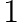’s in the position matching one of the distinct entries. Thus the only matrices with a chance of commuting are diagonal matrices of the form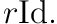Thus the map identifies the centrewith the centre ofProposition 5. If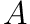is a central simple algebra over a field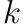, then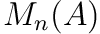is also a central simple algebra over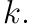By proposition 3 we see thatis simple iffis simple. By proposition 4 we see that we may identifywith the subring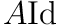ofand thathas center equal to the center of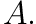Thus ifis a CSA overthen so is